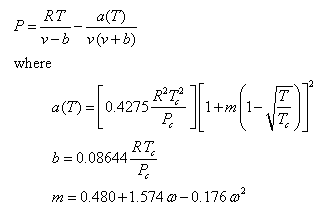Contents - Index

Introduction

The compressibility, enthalpy departure, entropy departure, and fugacity coefficient can all be calculated if an equation of state for a fluid is known.  The GEN_EOS routines use the Redlich-Kwong-Soave equation of state shown below. (Chem. Eng. Science, Vol. 27, pp. 1197-1203, 1972).    See the Thermodynamics book by Klein and Nellis for more information.The functions that can be called directly from EES are:

Compress(Tr, Pr, [w])
EnthDep(Tr, Pr, [w])
EntrDep(Tr, Pr, [w])
FugCoef(Tr, Pr, [w])

The inputs to all functions are Tr, Pr, and optionally, w where

Tr is the reduced temperature, i.e., the ratio of the temperature to critical temperature using an absolute temperature scale (dimensionless)

Pr is the reduced pressure, i.e., the ratio of the pressure to the critical pressure (dimensionless)

w is the acentric factor (dimensionless).  This parameter is optional. A default value of 0.1 is used if the third parameter is not provided.

The GEN_EOS.DLL was written and compiled in Embarcadero's DELPHI 10.  You may wish to view the source code to see how EES external functions are implemented.